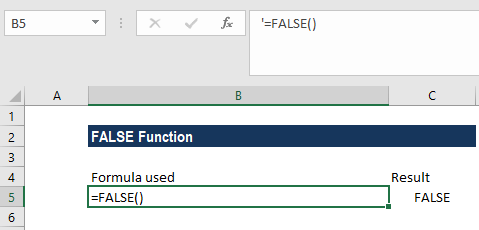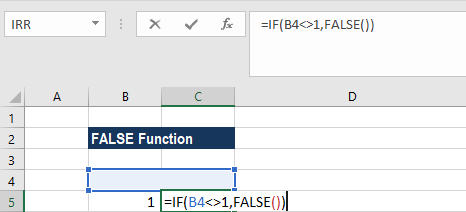# FALSE Function

Returns the logical value FALSE

## What is the FALSE Function?

The FALSE Function is categorized under Excel Logical functions. The function returns the logical value FALSE. It is as good as typing FALSE in an Excel sheet.

When doing financial analysis, this function becomes useful when we use it with the TRUE function. For example, we can use it if we wish to compare some cells. It can be used with other logical functions such as IF, IFERROR, etc. The function was introduced in Excel 2000 version and is available in all later versions.

### Formula

=FALSE( )

The FALSE function syntax does not require a parameter or argument. The function returns a value of FALSE.

### How to use the FALSE Function in Excel?

The FALSE function is a built-in function in Excel. To understand the uses of the function, let’s consider a few examples:

#### Example 1

Let’s consider this example:

If we type =FALSE() in an Excel cell as shown below, we get the result as FALSE.One can also enter the function directly into a cell. Even if we do not add the parentheses, it would return the Boolean value FALSE.

#### Example 2

Now, let’s consider another example:

Suppose we want the result as false if A1 is not equal to 1. The formula to use would be =IF(A1<>1,FALSE()). We will get the following result:We can also enter the value FALSE directly into cells and formulas without using this function. For example:

=IF(A1<>1, FALSE)

If the condition is met, Excel returns FALSE in both examples as specified by us. If the condition is not met, Excel returns TRUE in both examples.

Thanks for reading CFI’s guide to important Excel functions! By taking the time to learn and master these functions, you’ll significantly speed up your financial analysis. To learn more, check out these additional CFI resources:

• Excel Functions for Finance